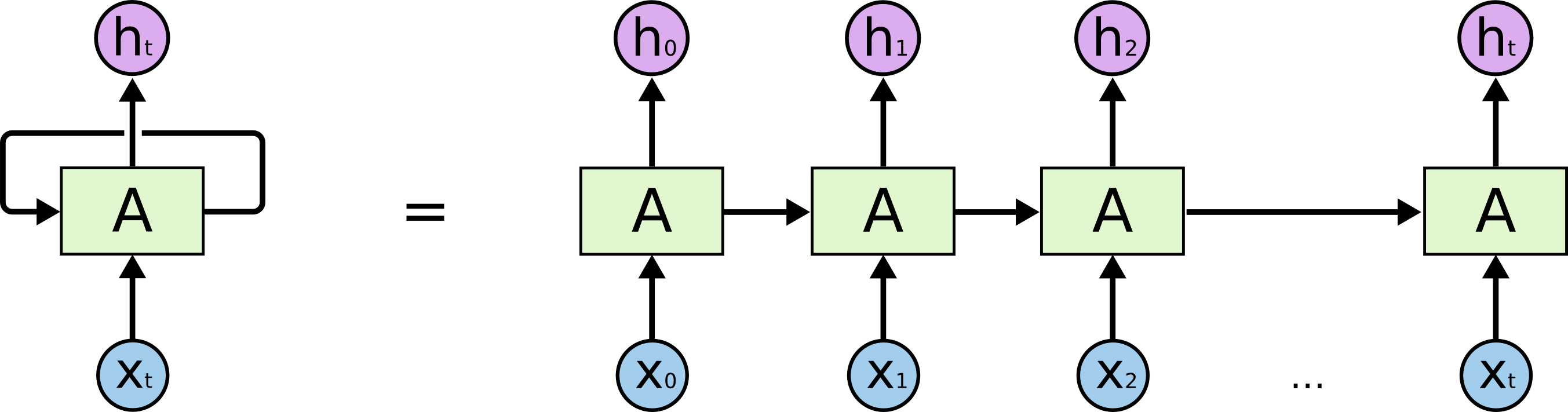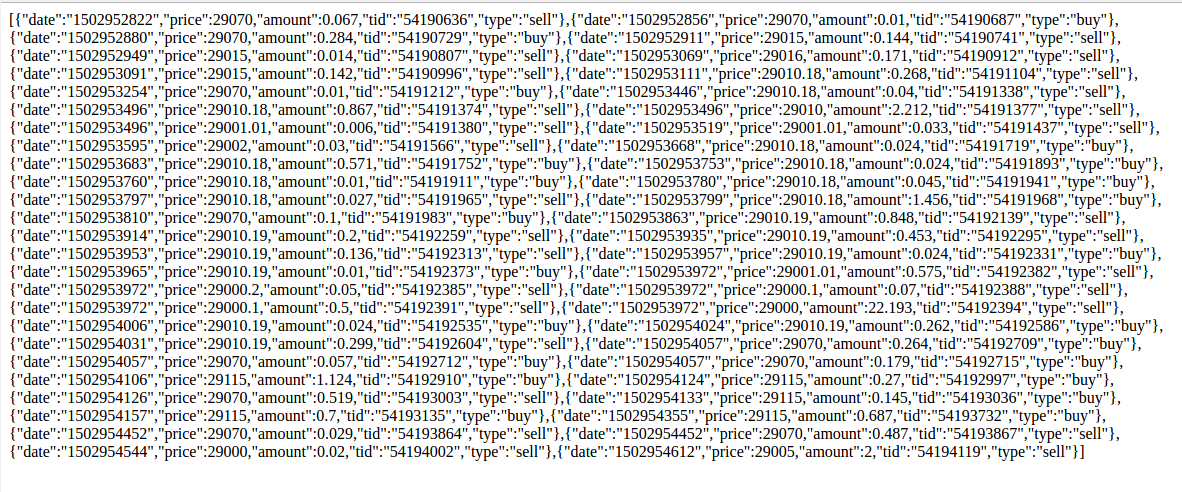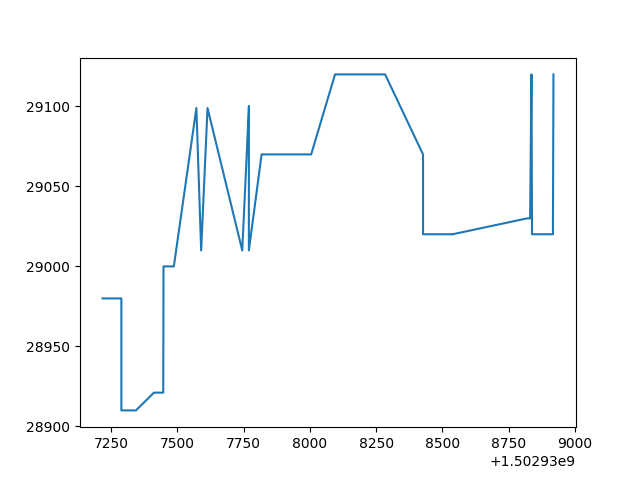# TensorFlow-Bitcoin-Robot:一个基于 TensorFlow LSTM 模型的 Bitcoin 价格预测机器人

## 简介

TensorFlow-Bitcoin-Robot:一个基于 TensorFlow LSTM 模型的 Bitcoin 价格预测机器人。LSTM（Long Short-Term Memory）是长短期记忆网络，是一种时间递归神经网络，适合于处理和预测时间序列中间隔和延迟相对较长的重要事件。LSTM 已经在科技领域有了多种应用。基于 LSTM 的系统可以学习翻译语言、控制机器人、图像分析、文档摘要、语音识别图像识别、手写识别、控制聊天机器人、预测疾病、点击率和股票、合成音乐等等任务。比特币的成交记录就是事件序列上的加个数据，可以基于过去的成交记录序列来对未来的价格作出预测。## 数据集[code lang=text]
pip install requests
pip install matplotlib
[/code]## 模型

rnn_predicter.py

[code lang=text]
for i in range(0,20):
#print(price)
one_predictor=np.array(price[i:i+20],dtype=float)
#print(one_predictor)
train_x.append(one_predictor)
if(int(price[i+20])>int(price[i+21])):
train_y.append(np.array([1,0,0]))
elif (int(price[i + 20]) == int(price[i + 21])):
train_y.append(np.array([0,1,0]))
elif(int(price[i+20])<int(price[i+21])):
train_y.append(np.array([0,0,1]))
[/code]

[code lang=text]
def RNN(x, weights, biases):
#首先把数据拆分为 n 个序列，每一个的维度 (batch_size, n_input)
x = tf.unstack(x, n_steps, 1)

# 定一个 lstm cell
lstm_cell = rnn.BasicLSTMCell(n_hidden, forget_bias=1.0)

# 获得 lstm 的输出
outputs, states = rnn.static_rnn(lstm_cell, x, dtype=tf.float32)
# 加个线性激活
return tf.matmul(outputs[-1], weights['out']) + biases['out']
[/code]

## 获得结果，定义损失函数和优化函数

[code lang=text]
pred = RNN(x, weights, biases)
# Define loss and optimizer
cost = tf.reduce_mean(tf.nn.softmax_cross_entropy_with_logits(logits=pred, labels=y))

# Evaluate model
correct_pred = tf.equal(tf.argmax(pred,1), tf.argmax(y,1))
accuracy = tf.reduce_mean(tf.cast(correct_pred, tf.float32))
[/code]

## 项目开源地址和训练结果

https://github.com/TensorFlowNews/TensorFlow-Bitcoin-Robot/

## 后续更新发布

http://www.tensorflownews.com/